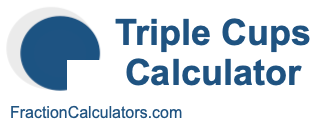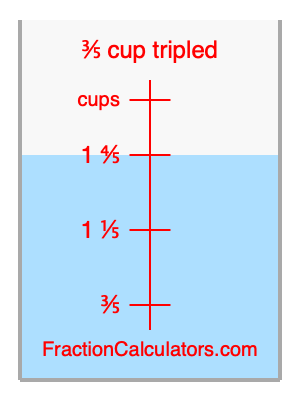What is 3/5 cup tripled?Here we will calculate 3/5 cup tripled and illustrate 3/5 cup tripled on a measuring cup. What is 3/5 cup tripled? 3/5 cup tripled is 3/5 cup times three. Therefore, to triple 3/5 cup, we multiply 3/5 by 3. Here is the formula, the math, and the answer to 3/5 cup tripled:

Cup × 3 = Cup tripled
3/5 × 3 = 1 4/5
3/5 cup tripled = 1 4/5 cups

Below is an illustration of a measuring cup. We show 3/5 cup and 3/5 cup tripled (1 4/5 cups) so you can see where they are in relation to each other.As you can see, we filled up our measuring cup to 3/5 cup tripled. In other words, we filled it up to 1 4/5, which is three times 3/5.

Triple Cups Calculator
Here you can triple another cup measurement.

What is cups tripled?

What is 5/8 cup tripled?
Here is the next measurement on our list that we have tripled for you.